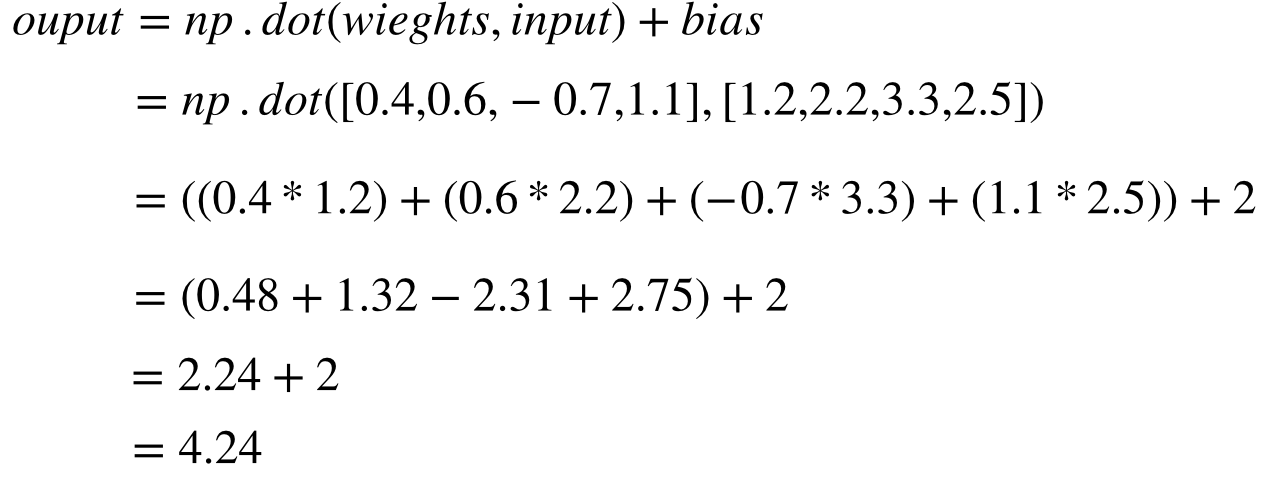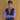# How to calculate dot product in perceptrons?

June 08, 2020

Neural networks are a collection of individuals nodes called as artificial neurons or perceptrons.

Each perceptron takes in an input array, multiples it with weights array and add a bias value to create a computed sum.

This computed computed is passed to an activation function to compute the final output value of a neuron.

We can see the basic parts of a perceptron below:

We can calculate the summation value of neuron inputs, weight and bias in the for a sample neuron as explained in example below:

``````#Example of dot product using numpy
import numpy as np

#Sample input to perceptron
inputs = [1.2, 2.2, 3.3, 2.5]

#Weights passed to perceptron
weights = [0.4,0.6,-0.7, 1.1]

#bias for a particular perceptron
bias = 2

#Take dot product between weights and input
#and add bias to the summation value
output = np.dot(weights, inputs) + bias
print(output)

#Output:-
4.24``````

Here np.dot function is used to calculate dot product between the input and the weights. Internally it works as follows:The output value from above function is fed to an activation function to calculate final value of a perceptron. I will cover various activation functions and their working in another article since it is a vast concept requiring it’s own article.

That’s it for today’s short tutorial on dot product using numpy.

Reference: Neural Networks from Scratch by SentDexBlog by Saurabh Mhatre
Follow me on Twitter